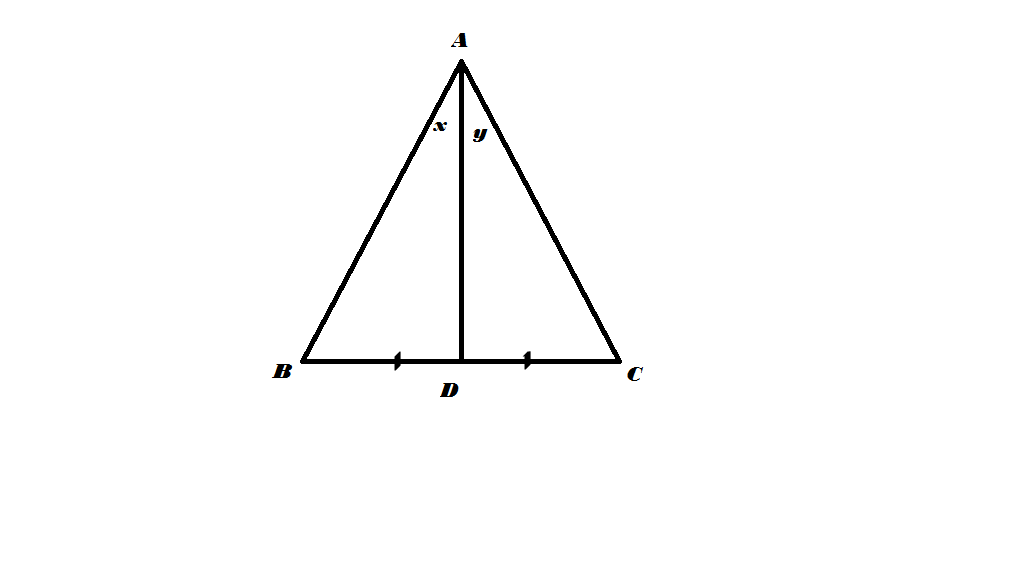# Jmetry1

Geometry Level 2In the above triangle ABC, AD is the median from A. Angle $x=45^\circ$ and angle $y=30^\circ$.Find the value of $\frac{AB}{AC}$?

Note:The answer is of the form $\frac{1}{\sqrt{a}}$.Enter the value of $a$.

×